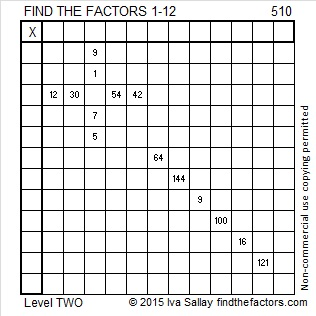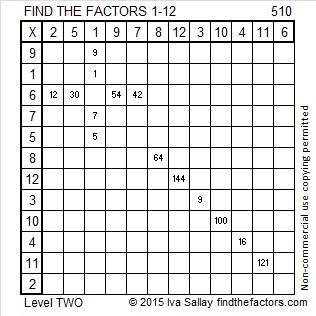# 510 and Level 2

510 is the sum of consecutive prime numbers three different ways.

1. 510 = 47 + 53 + 59 + 61 + 67 + 71 + 73 + 79
2. 510 = 31 + 37 + 41 + 43 + 47 + 53 + 59 + 61 + 67 + 71
3. 510 = 19 + 23 + 29 + 31 + 37 + 41 + 43 + 47 + 53 + 59 + 61 + 67

510 is the hypotenuse of four Pythagorean triples, none of which are primitive:

1. 78-504-510
2. 216-462-510
3. 240-450-510
4. 306-408-510

Two of those triples have the same greatest common factor. Can you identify which ones they are?Print the puzzles or type the solution on this excel file: 12 Factors 2015-06-01

—————————————————————————————————

• 510 is a composite number.
• Prime factorization: 510 = 2 x 3 x 5 x 17
• The exponents in the prime factorization are 1, 1, 1, and 1. Adding one to each and multiplying we get (1 + 1)(1 + 1)(1 + 1)(1 + 1) = 2 x 2 x 2 x 2 = 16. Therefore 510 has exactly 16 factors.
• Factors of 510: 1, 2, 3, 5, 6, 10, 15, 17, 30, 34, 51, 85, 102, 170, 255, 510
• Factor pairs: 510 = 1 x 510, 2 x 255, 3 x 170, 5 x 102, 6 x 85, 10 x 51, 15 x 34, or 17 x 30
• 510 has no square factors that allow its square root to be simplified. √510 ≈ 22.58317958—————————————————————————————————